Math Olympiad Test: Heron’s Formula- 1

# Math Olympiad Test: Heron’s Formula- 1

Test Description

## 10 Questions MCQ Test Mathematics Olympiad for Class 9 | Math Olympiad Test: Heron’s Formula- 1

Math Olympiad Test: Heron’s Formula- 1 for Class 9 2023 is part of Mathematics Olympiad for Class 9 preparation. The Math Olympiad Test: Heron’s Formula- 1 questions and answers have been prepared according to the Class 9 exam syllabus.The Math Olympiad Test: Heron’s Formula- 1 MCQs are made for Class 9 2023 Exam. Find important definitions, questions, notes, meanings, examples, exercises, MCQs and online tests for Math Olympiad Test: Heron’s Formula- 1 below.
 1 Crore+ students have signed up on EduRev. Have you?
Math Olympiad Test: Heron’s Formula- 1 - Question 1

### The area of a triangle, whose two sides are 8 cm and 11cm and the perimeter is 32 cm, will be:

Detailed Solution for Math Olympiad Test: Heron’s Formula- 1 - Question 1

Let s be the semi-perimeter and a, b and c are sides of a triangle
Using Heron's formula,
Area of triangle = √s(s – a)(s – b)(s – c)
a = 8 cm, b = 11 cm c = 32 - (8 + 11) = 13 cm
s = (8 + 11 + 13)/2 = 16
Area of triangle = √16(16 – 8)(16 – 11)(16 – 13)
⇒ Area of triangle = √16 × 8 × 5 × 3
⇒ √2 × 2 × 2 × 2 × 2 × 2 × 2 × 5 × 3
⇒ 2 × 2 × 2 × √30 =  8√30 cm2

Math Olympiad Test: Heron’s Formula- 1 - Question 2

### If the length of each side of a triangle is multiplied by 3, then the % increase in area will be:

Detailed Solution for Math Olympiad Test: Heron’s Formula- 1 - Question 2

Let the sides be a, b, c
∴ New sides = 3a, 3b, 3c
∴ snew = 3s
New area =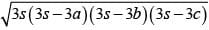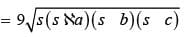= 9∆
∴ Increase in area = 9∆ – ∆ = 8∆
∴ % Increase in area =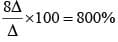Math Olympiad Test: Heron’s Formula- 1 - Question 3

### The sides of a triangle are 11cm, 15cm and 16 cm. The altitude to the largest side is:

Detailed Solution for Math Olympiad Test: Heron’s Formula- 1 - Question 3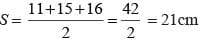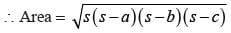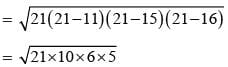= 5 × 2 × 3√7
=  √cm2
1/2 × Altitude to the largest side × largest side = 30√7 cm2
⇒ Altitude to the largest side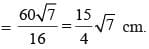Math Olympiad Test: Heron’s Formula- 1 - Question 4

The length of median of an equilateral triangle is √3 cm. The area of triangle is:

Detailed Solution for Math Olympiad Test: Heron’s Formula- 1 - Question 4

Length of median of equilateral triangle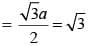⇒ a = 2 cm
∴ Area of triangle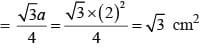Math Olympiad Test: Heron’s Formula- 1 - Question 5

In the figure, PQ : QR = 3 : 2. If the area of ∆PRT = 40 cm2, then area of ∆TQR is: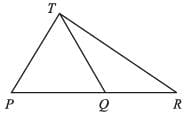Detailed Solution for Math Olympiad Test: Heron’s Formula- 1 - Question 5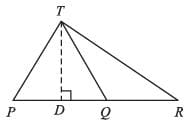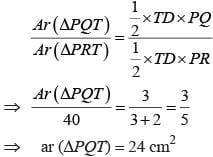⇒     ar (∆PQT) = 24 cm2
∴ area (∆TQR) = ar (∆PRT) – ar (∆PQT) = 40 – 24 = 16cm2

Math Olympiad Test: Heron’s Formula- 1 - Question 6

The area of an equilateral triangle with side 2√3 cm is

Detailed Solution for Math Olympiad Test: Heron’s Formula- 1 - Question 6

Given, the side of an equilateral triangle = 2√3 cm
We have to find the area of the equilateral triangle.
Area of an equilateral triangle = √3/4 (side)²
= √3/4 (2√3)²
= √3/4 (4 × 3)
= 3√3
Consider √3 = 1.732
= 3 × 1.732
= 5.196 cm²
Therefore, the area of an equilateral triangle is 5.196 cm²

Math Olympiad Test: Heron’s Formula- 1 - Question 7

If a square and equilateral triangle have same perimeter and, square has area A1 and equilateral triangle has area A2, then

Detailed Solution for Math Olympiad Test: Heron’s Formula- 1 - Question 7

Perimeter of square = Perimeter of equilateral ∆ = x
∴ length of side of square = x/4
Length of side of equilateral ∆ = x/3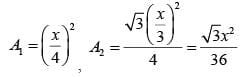Clearly, A1 > A2

Math Olympiad Test: Heron’s Formula- 1 - Question 8

Two parallel sides of a trapezium are 60 cm and 77 cm and other sides are 25 cm and 26 cm. The area of the trapezium is

Detailed Solution for Math Olympiad Test: Heron’s Formula- 1 - Question 8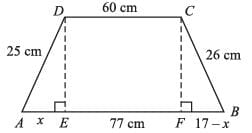AE = x, FB = (17 – x) cm
From ∆AED, and ∆CFB
CF2 = DE2
⇒ (26)2 – (17 – x)2 = (25)2 – (x)2
⇒ (26)2 – (25)2 = (17 – x)2 – (x)2
⇒ (26 – 25) (26 + 25) = (17 – x + x) (17 – x – x)
⇒ 51 = 17 (17 – 2x)
⇒ 17 – 2x = 3
⇒ 2x = 14 ⇒ x = 7cm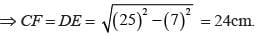∴ area = 1/2 x (60 + 77) x 24 cm2
= 137 × 12 cm2 = 1644 cm2

Math Olympiad Test: Heron’s Formula- 1 - Question 9

The area of rhombus whose perimeter is 80m and one of the diagonal is 24m.

Detailed Solution for Math Olympiad Test: Heron’s Formula- 1 - Question 9

Side of rhombus = 80/4 = 20 cm
∴ Length of other diagonal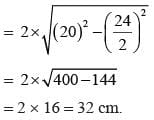∴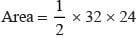= 16 × 24 cm2 = 384 cm2

Math Olympiad Test: Heron’s Formula- 1 - Question 10

Area of the figure is: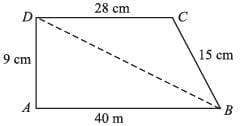Detailed Solution for Math Olympiad Test: Heron’s Formula- 1 - Question 10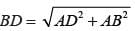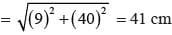∴ For ∆DBC,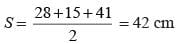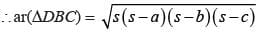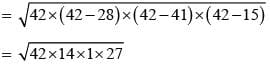= 14 × 3 × 3
= 126 cm2
Ar(∆ABD) = 1/2 x 9 x 40
= 180 cm2
∴ Total area = (126 + 180) cm2
= 306 cm2

## Mathematics Olympiad for Class 9

1 videos|43 tests
 Use Code STAYHOME200 and get INR 200 additional OFF Use Coupon Code